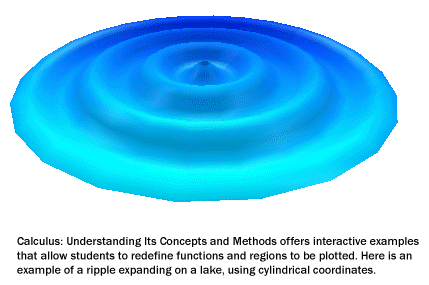# Calculus: Understanding Its Concepts and Methods

NOTE: Calculus: Understanding Its Concepts and Methods works only with Scientific Notebook 5.5 and runs on Windows®.Calculus: Understanding Its Concepts and Methods is a revolutionary CD-based calculus textbook that represents a dramatic change in the teaching and learning of calculus. It opens up a mathematical adventure with explanations, examples, explorations, problems, and self-tests that present calculus concepts and methods within a dynamic Scientific Notebook 5.5 environment.

### The Latest in Software Technology

The textbook is provided on CD and includes Version 5.5 of Scientific Notebook. With the powerful computer algebra system included in Scientific Notebook 5.5, students can interactively explore examples and carry out experiments that reveal what calculus is all about. The book exploits software technology to help students discover concepts interactively. Live calculations, animated plots, and manipulatable graphics and examples help clarify concepts, enhance the presentation of calculus concepts, and illustrate methods. The book is thoroughly indexed and hyperlinked to provide easy navigation and access to relevant information.

### An Ideal Teaching ToolCalculus: Understanding Its Concepts and Methods contains the information normally taught in a three-semester calculus sequence. Each chapter presents both basic concepts and computational details, and supplements explanations with tools, popup hints and information, examples, and explorations.

Many examples have animated mathematics that encourage student exploration, and some include step-by-step discussions of specific problem solutions. Others are interactive, so students can experiment by defining their own functions and parameters. The explorations challenge students to write detailed solutions to open-ended problems; some model solutions are included. Learning is further reinforced with extensive problem sets and algorithmically generated self-tests that allow students to measure their level of understanding.

With its focus on mathematical problem solving, experimentation, verification, and communication of results, Calculus: Understanding Its Concepts and Methods is an ideal teaching tool for classroom use, independent study, and distance learning.

### Support for Instructors and Students

Everything takes place in a Scientific Notebook 5.5 environment, which combines a scientific word processor with an easy-to-learn interface to an integrated computer algebra system. Students are encouraged to think and communicate like mathematicians, entering mathematics into documents using natural notation, not obscure programming syntax. The mathematics can be edited, evaluated, plotted, simplified, factored, and expanded, and the output, also in natural notation, can be used in subsequent computations.

In addition to illustrated explanations, animated examples, and opportunities for exploration, the textbook supports the instructor with hints to the student about how to approach problems, extensive problem sets with complete answers, and additional resource material. Self-tests in each chapter are randomly generated from problem prototypes, providing a virtually unlimited number of distinct quizzes to test students' understanding.

Students with questions about calculus can participate in an unmoderated discussion forum for users of Calculus: Understanding Its Concepts and Methods. And they can get fast answers to questions about using the tools and features in Scientific Notebook 5.5 from the help files in each chapter and the program's online Help system.